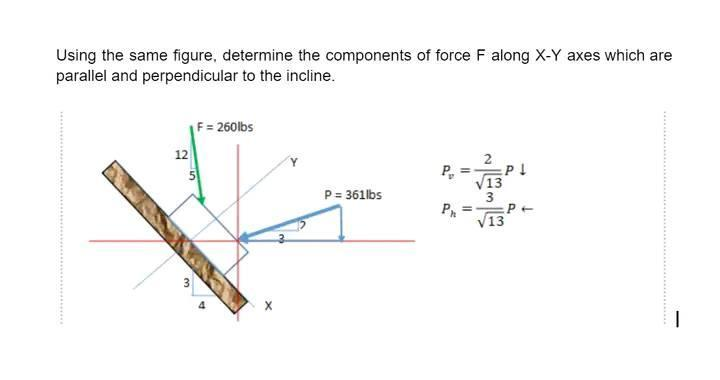Home / Expert Answers / Civil Engineering / using-the-same-figure-determine-the-components-of-force-p-nbsp-along-xy-axes-which-are-parallel-pa532

# (Solved): Using the same figure, determine the components of force P along XY axes which are parallel ...

Using the same figure, determine the components of force P along X?Y axes which are parallel and perpendicular to the incline.Using the same figure, determine the components of force $$F$$ along $$X-Y$$ axes which are parallel and perpendicular to the incline. $\begin{array}{l} P_{v}=\frac{2}{\sqrt{13}} P \downarrow \\ P_{h}=\frac{3}{\sqrt{13}} P \leftarrow \end{array}$

We have an Answer from Expert